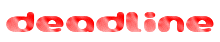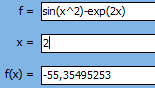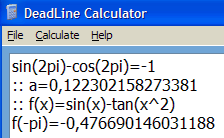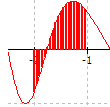DeadLine - analyze, solve, and plot various mathematical equations.

## Do you solve equations and plot graphs often?

You have Math homeworks, engineering projects or you just like to understand more about Math. Then you know it's not easy to find equation roots. If you use DeadLine, a simple yet powerful equation solver, your results will be precise and ready to use. Visualize equation graphs in full screen and understand what lies behind a cryptic Math equation.

DeadLine is a freeware designed for students and engineers. It combines graph plotting with advanced numerical Calculus, in a very intuitive approach. Most equations are supported, including algebraic equations, trigonometric equations, and exponential equations.

DeadLine includes a fast engine that performs Math calculations. You can complete any task almost instantly, even on older systems.

## How can you use DeadLine?

solve equations
plot graphs interactively
perform calculations
derive functions and evaluate derivatives
solve optimization problems: find local extrema
integrate functions numerically
solve parametric equations
obtain an in-depth analysis of a function
save results: equations, graphs, roots and extrema
solve Calculus assignments in your own language

## Evaluate functions accurately.Evaluate functions and the first two derivatives as you type. DeadLine recognizes the most used Math functions: trigonometric (sine, cosine, tangent, inverse sine, inverse cosine, inverse tangent), hyperbolic (hyperbolic sine, hyperbolic cosine, hyperbolic tangent, inverse hyperbolic sine, inverse hyperbolic cosine, inverse hyperbolic tangent), natural logarithm, exponential, square root, absolute value. DeadLine supports "implicit multiplication", so you can simply enter 2sin(x^2)(ln(abs(x))+1). The evaluation point will be automatically displayed on the graph.## Derive functions and find local extrema.

Find the first two derivatives in a symbolic form, plot derivatives' graph, find the extreme points (local maxima and minima) and solve optimization problems. Derivatives are useful for examining the graphs of functions. In particular, the points in the interior of the domain of a function which take that function to local extrema will all have a first derivative of zero.## Calculate the integral.

Numerical integration computes an approximation of the integral over some interval. The integral value is displayed as you type the interval and the integration area is shaded on the graph.

## Plot graphs interactively.

Plot graphs, zoom in or zoom out to narrow / widen the scope, while remaining centered. Click outside the graph, to move the graph in any direction, Adjust the graph domain defining the bounds explicitly. Customize graph properties to suit your needs: change the precision of the graph, modify the color of the graph, axes and grid, or choose a new graph title.

## Solve algebraic equations.

While there is no flawless technique to solve equations, this educational software combines the most successful numerical methods, in order to deliver the right answer. Many equations cannot be solved analytically, that's why other methods of solving equations are needed, including graphical and numerical method. DeadLine Equation Solver automatically displays the real roots of an equation in a list so you can mark each root on the graph.

## Most equations are supported.

• algebraic equations
• trigonometric equations
• exponential equations
• logarithmic equations

## Export the graph and save the results.

If you want to include the graph, the extrema or the roots of an equation in your documents, you have the option to export them. The graphs can be exported in four high-quality formats: GIF, PNG, TIFF, and BMP. You can also copy the graphs to the clipboard in order to use them in other applications (for example, in a Microsoft Word document). Take a look at an exponential equation solved by DeadLine. Try to solve the equation by hand and then compare your results to DeadLine's Math homework.

## Get context sensitive help and online support.

For more details about solving equations with DeadLine, use the built-in help, which includes step-by-step descriptions of the features. Unlike other educational software, DeadLine provides free online support: if you have questions or suggestions, visit DeadLine Support Center.